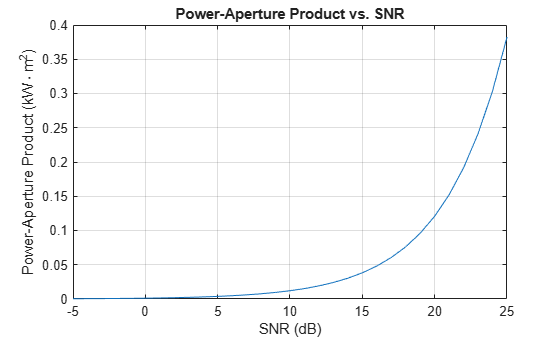Power-aperture product using search radar equation

## Syntax

``pap = radareqsearchpap(range,snr,omega,tsearch)``
``pap = radareqsearchpap(___,Name,Value)``

## Description

````pap = radareqsearchpap(range,snr,omega,tsearch)` computes the available power-aperture product, `pap`, for a surveillance radar based on the range, `range`, required signal-to-noise ratio (SNR), `snr`, solid angular search volume, `omega`, and search time, `tsearch`.```

example

````pap = radareqsearchpap(___,Name,Value)` computes the available power-aperture product with additional options specified by one or more name-value arguments. For example, `'Loss',6` specifies system losses as 6 decibels.```

## Examples

collapse all

Compute the power-aperture product for a search radar that is required to detect a 1 square meter RCS target at a range of `111` kilometers. Assume the antenna rotates at a rate of `12.5` RPM, the signal-to-noise ratio required to make a detection is `13` decibels, the system noise temperature is `487` Kelvin, and the total system loss is `20` decibels.

```range = 111e3; tsearch = 60 / 12.5; snr = 13; ts = 487; loss = 20;```

The radar traverses a search volume with azimuths in the range [–180,180] degrees and elevations in the range [0,45] degrees. Find the solid angular search volume in steradians by using the `solidangle` function.

```az = [-180;180]; el = [0;45]; omega = solidangle(az,el);```

Calculate the power-aperture product. By default, the target RCS is 1 square meter.

`snr = radareqsearchpap(range,snr,omega,tsearch,'Ts',ts,'Loss',loss)`
```snr = 2.3689e+04 ```

Plot the power-aperture product as a function of the required SNR for a search radar system located at a range of `100` kilometers. Incorporate path loss due to absorption into the calculation of the power-aperture product.

Specify the required SNR as values in the range [–5,25] decibels. Assume the search volume is `1.5` steradians and the search time is `12` seconds.

```range = 100e3; snr = -5:25; omega = 1.5; tsearch = 12;```

Find the path loss due to atmospheric gaseous absorption by using the `gaspl` function. Specify the radar operating frequency as `10` GHz, the temperature as `15` degrees Celsius, the dry air pressure as `1013` hPa, and the water vapour density as `7.5` $\mathrm{g}/{\mathrm{m}}^{3}$.

```freq = 10e9; temp = 15; pressure = 1013e2; density = 7.5; loss = gaspl(range,freq,temp,pressure,density);```

Compute the power-aperture product. By default, the target RCS is 1 square meter.

`pap = radareqsearchpap(range,snr,omega,tsearch,'AtmosphericLoss',loss);`

Plot the power-aperture product as a function of the required SNR. Before plotting, convert the power-aperture product from $\mathrm{W}\cdot {\mathrm{m}}^{2}$ to $\mathrm{kW}\cdot {\mathrm{m}}^{2}$.

```plot(snr,pap*0.001) grid on xlabel('SNR (dB)') ylabel('Power-Aperture Product (kW\cdotm^2)') title('Power-Aperture Product vs. SNR')```## Input Arguments

collapse all

Range, specified as a scalar or a length-J vector of positive values, where J is the number of range samples. Units are in meters.

Example: `1e5`

Data Types: `double`

Required signal-to-noise ratio (SNR), specified as a scalar or a length-J vector of real values. Units are in decibels.

Example: `13`

Data Types: `double`

Solid angular search volume, specified as a scalar. Units are in steradians.

Given the elevation and azimuth ranges of a region, you can find the solid angular search volume by using the `solidangle` function.

Example: `0.3702`

Data Types: `double`

Search time, specified as a scalar. Units are in seconds.

Example: `10`

Data Types: `double`

### Name-Value Arguments

Specify optional pairs of arguments as `Name1=Value1,...,NameN=ValueN`, where `Name` is the argument name and `Value` is the corresponding value. Name-value arguments must appear after other arguments, but the order of the pairs does not matter.

Before R2021a, use commas to separate each name and value, and enclose `Name` in quotes.

Example: `'Ts',487` specifies the system noise temperature as 487 Kelvin

Radar cross section of the target, specified as a positive scalar or length-J vector of positive values. The `radareqsearchpap` function assumes the target RCS is nonfluctuating (Swerling case 0). Units are in square meters.

Data Types: `double`

System noise temperature, specified as a positive scalar. Units are in Kelvin.

Data Types: `double`

System losses, specified as a scalar or a length-J vector of real values. Units are in decibels.

Example: `1`

Data Types: `double`

One-way atmospheric absorption loss, specified as a scalar or a length-J vector of real values. Units are in decibels.

Example: `[10,20]`

Data Types: `double`

One-way propagation factor for the transmit and receive paths, specified as a scalar or a length-J vector of real values. Units are in decibels.

Example: `[10,20]`

Data Types: `double`

Custom loss factors, specified as a scalar or a length-J vector of real values. These factors contribute to the reduction of the received signal energy and can include range-dependent sensitivity time control (STC), eclipsing, and beam-dwell factors. Units are in decibels.

Example: `[10,20]`

Data Types: `double`

## Output Arguments

collapse all

Power-aperture product, returned as a scalar or a length-J column vector of positive values, where J is the number of range samples. Units are in W·m2.

Data Types: `double`

collapse all

### Power-Aperture Product Form of Search Radar Equation

The power-aperture product form of the search radar equation, PavA, is:

`${P}_{av}A=\frac{4\pi \Omega {R}^{4}k{T}_{s}\left(SNR\right){L}_{a}^{2}L}{{t}_{s}\sigma {F}^{2}{F}_{c}}$`

where the terms of the equation are:

• Ω — Search volume in steradians

• R — Target range in meters. The equation assumes the radar is monostatic

• k — Boltzmann constant

• Ts — System temperature in Kelvin

• SNR — Required signal-to-noise ratio

• La — One-way atmospheric absorption loss

• L — Combined system losses

• ts — Search time in seconds

• σ — Nonfluctuating target radar cross section in square meters

• F — One-way propagation factor for the transmit and receive paths

• Fc — Combined range-dependent factors that contribute to the reduction of the received signal energy

You can derive this equation by rearranging the SNR form of the search radar equation. See the `radareqsearchsnr` function for more information.

 Barton, David Knox. Radar Equations for Modern Radar. Artech House Radar Series. Boston, Mass: Artech House, 2013.

 Skolnik, Merrill I. Introduction to Radar Systems. Third edition. McGraw-Hill Electrical Engineering Series. Boston, Mass. Burr Ridge, IL Dubuque, IA: McGraw Hill, 2001.